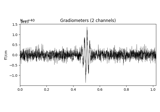# mne.time_frequency.tfr_array_morlet¶

mne.time_frequency.tfr_array_morlet(epoch_data, sfreq, freqs, n_cycles=7.0, zero_mean=False, use_fft=True, decim=1, output='complex', n_jobs=1, verbose=None)[source]

Compute Time-Frequency Representation (TFR) using Morlet wavelets.

Same computation as `tfr_morlet`, but operates on `NumPy arrays` instead of `Epochs` objects.

Parameters
epoch_data`array` of shape (n_epochs, n_channels, n_times)

The epochs.

sfreq

Sampling frequency of the data.

freqsarray_like of `float`, shape (n_freqs,)

The frequencies.

n_cycles`float` | `array` of `float`, default 7.0

Number of cycles in the Morlet wavelet. Fixed number or one per frequency.

zero_mean

If True, make sure the wavelets have a mean of zero. default False.

use_fftbool

Use the FFT for convolutions or not. default True.

decim

To reduce memory usage, decimation factor after time-frequency decomposition. default 1 If `int`, returns tfr[…, ::decim]. If `slice`, returns tfr[…, decim].

Note

Decimation may create aliasing artifacts, yet decimation is done after the convolutions.

output`str`, default ‘complex’
• ‘complex’ : single trial complex.

• ‘power’ : single trial power.

• ‘phase’ : single trial phase.

• ‘avg_power’ : average of single trial power.

• ‘itc’ : inter-trial coherence.

• ‘avg_power_itc’ : average of single trial power and inter-trial coherence across trials.

n_jobs`int`

The number of jobs to run in parallel (default 1). Requires the joblib package. The number of epochs to process at the same time. The parallelization is implemented across channels. Default 1.

verbose

If not None, override default verbose level (see `mne.verbose()` and Logging documentation for more). If used, it should be passed as a keyword-argument only.

Returns
out`array`

Time frequency transform of epoch_data. If output is in [‘complex’, ‘phase’, ‘power’], then shape of out is (n_epochs, n_chans, n_freqs, n_times), else it is (n_chans, n_freqs, n_times). If output is ‘avg_power_itc’, the real values code for ‘avg_power’ and the imaginary values code for the ‘itc’: out = avg_power + i * itc.

Notes

New in version 0.14.0.

## Examples using `mne.time_frequency.tfr_array_morlet`¶